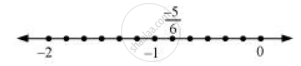Share

# Represent These Numbers on the Number Line. -5by6 - CBSE Class 8 - Mathematics

ConceptRepresentation of Rational Numbers on the Number Line

#### Question

Represent these numbers on the number line.

-5/6

#### Solution

-5/6 can be represented on the number line as follows.Is there an error in this question or solution?

#### APPEARS IN

NCERT Solution for Mathematics Textbook for Class 8 (2018 to Current)
Chapter 1: Rational Numbers
Ex. 2.10 | Q: 1.2 | Page no. 20

#### Video TutorialsVIEW ALL 

Solution Represent These Numbers on the Number Line. -5by6 Concept: Representation of Rational Numbers on the Number Line.
S